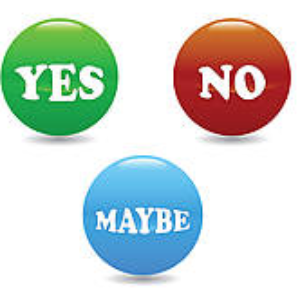# Opinion Polls: Delphi's Polling Place

Hosted by Showtalk

Opinion polls on all subjects. Opinions? Heck yes, we have opinions - but we're *always* nice about it, even when ours are diametrically opposed to yours. Register your vote today!

• 4583
MEMBERS
• 101626
MESSAGES
• 16
POSTS TODAY

# What is it about the number 3 and mathem

Started 10/19/21 by WALTER784; 209 views.#### From: WALTER784

10/19/21

What is it about the number 3 and mathematics magic?

 # x 3 + 3 x 3 -> "Left Digit" + "Right Digit" = Ans 1 3 6 18 -> 1 + 8 = 9 2 6 9 27 -> 2 + 7 = 9 3 9 12 36 -> 3 + 6 = 9 4 12 15 45 -> 4 + 5 = 9 5 15 18 54 -> 5 + 4 = 9 6 18 21 63 -> 6 + 3 = 9 7 21 24 72 -> 7 + 2 = 9 8 24 27 81 -> 8 + 1 = 9 9 27 30 90 -> 9 + 0 = 9

 # x 3 . x 3 -> "Left Digit" + "Right Digit" = Ans 1 3 . 9 -> 0 + 9 = 9 2 6 . 18 -> 1 + 8 = 9 3 9 . 27 -> 2 + 7 = 9 4 12 . 36 -> 3 + 6 = 9 5 15 . 45 -> 4 + 5 = 9 6 18 . 54 -> 5 + 4 = 9 7 21 . 63 -> 6 + 3 = 9 8 24 . 72 -> 7 + 2 = 9 9 27 . 81 -> 8 + 1 = 9

They all strangely add up to the number 9 regardless of whether addition and multiplication or just multiplication are used.

FWIW